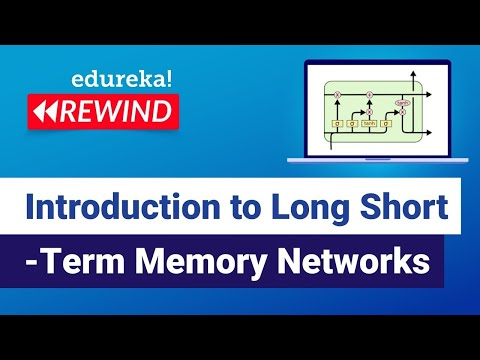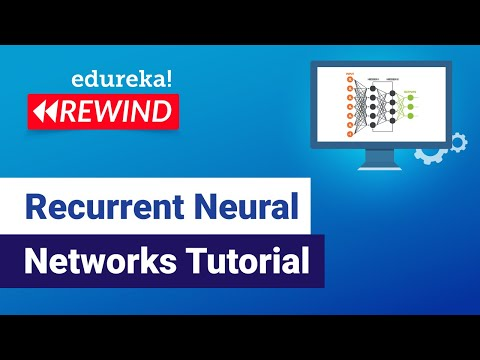# recurrent neural network tutorialNeural Networks

#### Introduction to Long Short-Term Memory Networks (LSTM)

This Edureka LSTM Explained video will help you in understanding why we need Recurrent Neural Networks (RNN) and what exactly it is. It also explains few issues with training a Recurrent Neural Network and how to overcome those challenges using LSTMs.Neural Networks

#### Recurrent Neural Networks Tutorial

This Edureka Recurrent Neural Networks tutorial video will help you in understanding why we need Recurrent Neural Networks (RNN) and what exactly it is. It also explains few issues with training a Recurrent Neural Network and how to overcome those challenges using LSTMs. The last section includes a use-case of LSTM to predict the next […]